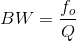# Calculate 3dB Bandwidth and Cutoff Frequency

Calculator finds 3dB Bandwidth and Cutoff Frequency for selected values.

 Enter Center Frequency: (f0) Hz Enter Quality Factor (Q):
 Lower cut off frequency (f1)= Hz Upper cut off frequency (f2)= Hz 3 dB Bandwidth (BW)= Hz
A cutoff frequency is a border in a system's frequency response at which energy flowing through the system starts to decrease or reflected without being transmitted.
Formula:Where,
BW=Bandwidth of a circuit in unit of frequency,
fo=center frequency,
f2=the upper cut off frequency,
f1=the lower cut off frequency